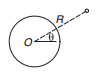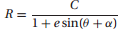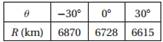Create an Account

Home / Questions / The trajectory of a satellite orbiting the earth is where R θ are the polar coordinate...

The trajectory of a satellite orbiting the earth is where R θ are the polar coordinates of the satellite and C e and α are constants e is known as the eccentricity of the orbit). If the sateThe trajectory of a satellite orbiting the earth iswhere (R, θ) are the polar coordinates of the satellite, and C, e and α are constants (e is known as the eccentricity of the orbit). If the satellite was observed at the following three positionsdetermine the smallest R of the trajectory and the corresponding value of θ.

Jul 27 2020 View more View LessSubscribe To Get Solution